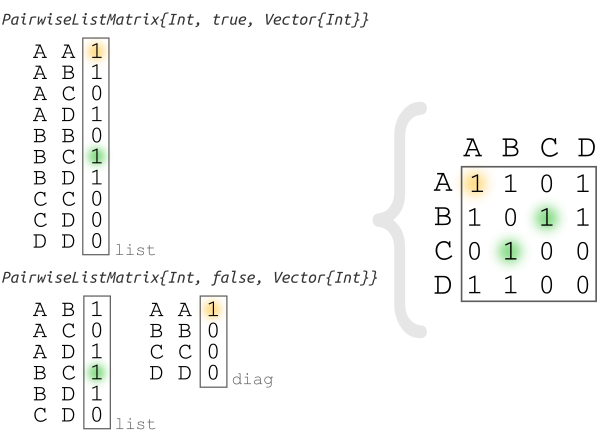## PairwiseListMatrices.jl

Symmetric matrix as a list of the values in its upper or lower triangular part. Useful for representing pairwise measures or comparisons when the used metric or distance satisfies the symmetry condition and for adjacency matrices of undirected graphs.
Author diegozea
Popularity
13 Stars
Updated Last
2 Years Ago
Started In
September 2015

# PairwiseListMatrices

## Description

This package allows you to use a pairwise list as a matrix:```mutable struct PairwiseListMatrix{T,diagonal,VT} <: AbstractArray{T, 2}
list::VT
diag::VT
nelements::Int
...
end```

`PairwiseListMatrix{T, diagonal, VT}` is a (squared) symmetric matrix that stores a `list` of type `VT` with values of type `T` for the pairwise comparison/evaluation of `nelements`. If `diagonal` is `true` the first element of the list is `1, 1` otherwise is `1, 2`. If `diagonal` is `false` the diagonal values are stored in a vector on the `diag` field.

## Features

#### Space

In pairwise calculations like `cor()` if results are saved as `PairwiseListMatrix` the space is `N(N+1)/2` instead of `N*N`. This is useful to compare a large number of elements, because you are saving ~ 50% of the memory.

#### Time

`PairwiseListMatrix` is faster than a full matrix to make operatation like `sum` and `mean` in the whole matrix, since it is cache efficient. However it is slower than a full matrix for reducing along dimensions.

## Example

```julia> # Pkg.add("PairwiseListMatrices")

julia> using PairwiseListMatrices

julia> plm  = PairwiseListMatrix([1,2,3], false)
3×3 PairwiseListMatrices.PairwiseListMatrix{Int64,false,Array{Int64,1}}:
0  1  2
1  0  3
2  3  0

julia> nplm  = setlabels(plm, ["a","b","c"])
3×3 Named PairwiseListMatrices.PairwiseListMatrix{Int64,false,Array{Int64,1}}
A ╲ B │ a  b  c
──────┼────────
a     │ 0  1  2
b     │ 1  0  3
c     │ 2  3  0

julia> to_table(nplm)
6×3 Array{Any,2}:
"a"  "a"  0
"a"  "b"  1
"a"  "c"  2
"b"  "b"  0
"b"  "c"  3
"c"  "c"  0
```